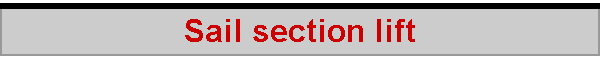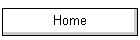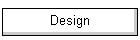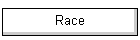Here is a simple theory of how a sail develops the lift it does. We start with a flat plate, and point it into the wind.  Not a lot happens, of course -- it gives no lift.  If we put some curve into the plate, giving it some camber, an interesting thing happens -- it develops quite considerable lift.  In the example, the plate has 10% draft, and it develops a coefficient of lift of around 0.90.  Notice that the cambered plate is not developing any angle of attack.In theory, the lift coefficient at zero angle of attack is 4 pi times the camber for a circular arc plate -- about 1.26 for our 10% cambered plate.  This overestimates the zero alpha lift coefficient for 3-D, finite span foils.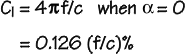Let's see if we can get some lift out of our flat plate.  Of course we can, by tilting it into the wind.  Giving it an angle of attack like this allows the flat plate to reach a coefficient of lift of around 0.8, maximum, and it reaches this maximum lift at an angle of attack of around 8 degrees.  We can do even better with our cambered plate.  When we tilt it into the wind, it can develop a maximum coefficient of lift of around 1.75, at an angle of attack of about 13 degrees.  (In both cases, the incident wind shows some upwash as it approaches the leading edge.)We've learned a couple of things.  Cambering the plate allows it to develop more lift.  Remember that a flat plate is pretty good at developing lift, but it runs out of capability quite quickly at a lift coefficient of around 0.8.  Cambering the plate allows a maximum coefficient of lift of 1.75, around 0.95 above and beyond a flat plate.  Part of the reason it can do this is that the cambering allows the plate to reach a higher angle of attack before stalling, because the leading edge of the plate can point into the wind better. The other thing we've learned is that a cambered plate, when laid flat, develops some lift anyway, just because it is cambered.  In our example, the lift coefficient was 0.9.  Hang on, this is pretty similar to the additional lift it develops over and above a flat plate when angled into the wind.  Turns out that these two coefficients are the same, and the additional lift of a cambered plate over a flat plate is pretty much a constant, due to its camber.  So you can add the coefficient of lift due to camber to the maximum coefficient of lift shown by a flat plate. If we had a cambered plate with, say, 5% draft, and found that, when laid flat, it developed a coefficient of lift due to its camber of 0.5, then we could say immediately that it's maximum coefficient of lift would be around 0.5 + 0.8 = 1.3 -- which is exactly right. An interesting question is, at what angle of attack does the cambered plate develop no lift at all?  This is called the "zero lift" angle of attack, and is negative for a cambered plate.  For our 10% cambered plate, this is around -5 degrees.  (The data here and elsewhere on this page comes from Marchaj's Aero-hydrodynamics Fig 2-70.  Marchaj says this data is for 2-D flow, but I'm not convinced, it has 3-D features all over it.  Nevertheless, it is the only systematic published data for sail-like shapes I've found so far, and the general trends it reports seem quite correct.)The zero lift angle of attack is theoretically important because it is the point at which the cambered plate begins to develop lift.  Many "standard" texts on aerodynamics tell you that lift "begins" when you angle your wing into the wind, but this isn't strictly true.  Our 10% cambered plate, by the time it points into the wind at no angle of attack, has in fact been developing lift for the previous 5 degrees of angle, and reaches a lift coefficient of 0.9 in the process. In theory, the angle of zero lift for a cambered circular arc plate is: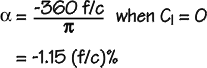This theoretical formula seems to apply to "potential", 2-D flow, and overestimates zero lift alpha for 3-D, finite span foils.  According to this formula, our 10% camber plate should show zero lift at around -11.5 degrees, but according to Marchaj's data, it does this at around -5 degrees.  One of the many little discrepancies between theory and experiment, perhaps. The cambered plate goes on to develop more lift, eventually reaching a maximum lift coefficient of about 1.75 at an angle of attack of 13 degrees.  But it started developing lift from an angle of attack of -5 degrees, and so has spent around 13 + 5 = 18 degrees developing its lift coefficient to a maximum of 1.75.  It turns out that this is another constant -- most aerofoils develop about 0.1 lift coefficient per degree of attack, counting from zero lift.  The flat plate developed a lift coefficient of 0.8 over 8 degrees, starting from zero lift.  Yup, 0.1 lift coefficient per degree of attack.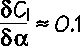2018-06-22